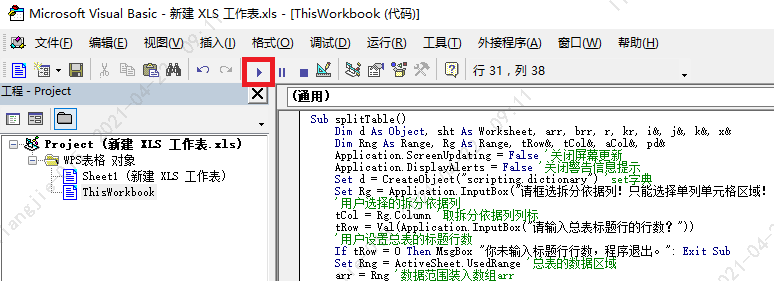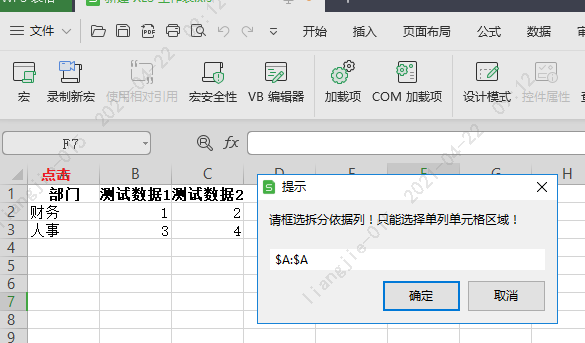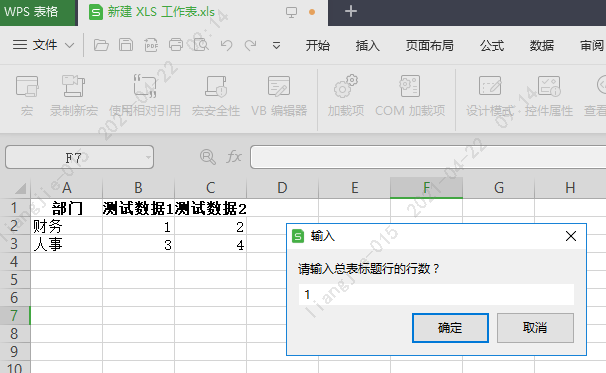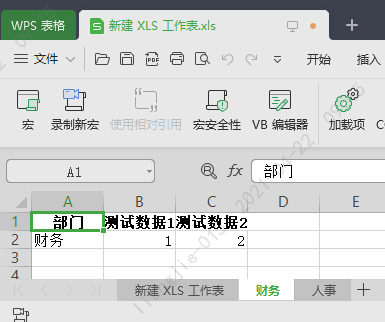• 一个很好地可以用做VB存放数据到EXCEL表格文件的样例
• VB FlexCell控件生成Excel表格效果，FlexCell表格制作系统，自学VB一年有余，深知编程之苦之乐，有时为某一功能的实现要花费许多时间，概因周围无可交流人员。为使后学者在某些方面少走弯路，特制作此软件，并公布源...
• VB6导出到Excel的三种方法全部编译通过，可以放心下载，文字描述较少，基本都是代码。VB Excel
• 内容索引:C#源码,报表打印,Excel　C#调用OFFICE组件生成Excel表格示例，注意本程序需要以下OFFICE组件，这些组件要求你必须装有OFFICE2003及以上版本，因为这结版本中才有微软可编程再生库资源，尤其是下列几个控件...
• 金蝶K3凭证生成 [VB6源码] 设置Excel表格自动生成凭证 项目核算自动生成,已在金蝶K3 10.4版及金蝶 K3 RISE V12.2上测试通过
• 利用VB操作EXCEL生成复杂的报表 vb操作EXCEL文件_编程技术收藏
• VB生成Excel

2013-03-25 00:13:22
VB 生成Excel,里面包含了许多表格画线的操作，作流程图的好东西VB Excel
• 其实现在有些版本的excel或wps已经集成了根据某列数据自动拆分表格数据，不过有些老版本的仍不支持，搜集了网上前辈的资料，结合自身实际，使用VB实现了该功能。 Sub splitTable() Dim d As Object, sht As ...

工作场景：从系统导出后人工处理完的excel数据，需要按照部门拆分并推送给各部门人员。

传统的工作方式就只能人工一个个部门筛选，复制粘贴出来各自发送。

其实现在有些版本的excel或wps已经集成了根据某列数据自动拆分表格数据，不过有些老版本的仍不支持，搜集了网上前辈的资料，结合自身实际，使用VB实现了该功能。

Sub splitTable()
Dim d As Object, sht As Worksheet, arr, brr, r, kr, i&, j&, k&, x&
Dim Rng As Range, Rg As Range, tRow&, tCol&, aCol&, pd&
Application.ScreenUpdating = False '关闭屏幕更新
Set d = CreateObject("scripting.dictionary") 'set字典
Set Rg = Application.InputBox("请框选拆分依据列！只能选择单列单元格区域！", Title:="提示", Type:=8)
'用户选择的拆分依据列
tCol = Rg.Column '取拆分依据列列标
tRow = Val(Application.InputBox("请输入总表标题行的行数？"))
'用户设置总表的标题行数
If tRow = 0 Then MsgBox "你未输入标题行行数，程序退出。": Exit Sub
Set Rng = ActiveSheet.UsedRange '总表的数据区域
arr = Rng '数据范围装入数组arr
tCol = tCol - Rng.Column + 1 '计算依据列在数组中的位置
aCol = UBound(arr, 2) '数据源的列数
For i = tRow + 1 To UBound(arr) '遍历数组arr
If Not d.exists(arr(i, tCol)) Then
d(arr(i, tCol)) = i '字典中不存在关键词则将行号装入字典
Else
d(arr(i, tCol)) = d(arr(i, tCol)) & "," & i '如果存在则合并行号，以逗号间隔
End If
Next
For Each sht In Worksheets '遍历一遍工作表，如果字典中存在则删除
If d.exists(sht.Name) Then sht.Delete
Next
kr = d.keys '字典的key集
For i = 0 To UBound(kr) '遍历字典key值
If kr(i) <> "" Then '如果key不为空
r = Split(d(kr(i)), ",") '取出item里储存的行号
ReDim brr(1 To UBound(r) + 1, 1 To aCol) '声明放置结果的数组brr
k = 0
For x = 0 To UBound(r)
k = k + 1 '累加记录行数
For j = 1 To aCol '循环读取列
brr(k, j) = arr(r(x), j)
Next
Next
'新建一个工作表，位置在所有已存在sheet的后面
.Name = kr(i) '表格命名
.[a1].Resize(tRow, aCol) = arr '放标题行
.[a1].Offset(tRow, 0).Resize(k, aCol) = brr '放置数据区域
Rng.Copy '复制粘贴总表的格式
.[a1].PasteSpecial Paste:=xlPasteFormats, Operation:=xlNone, SkipBlanks:=False, Transpose:=False
.[a1].Select
End With
End If
Next
Sheets(1).Activate '激活第一个表格
Set d = Nothing '释放字典
Erase arr: Erase brr '释放数组
MsgBox "数据拆分完成！"
Application.ScreenUpdating = True '恢复屏幕更新

'开始生成新excel文件
Application.ScreenUpdating = False
For Each sht In Worksheets
sht.Copy
ActiveWorkbook.SaveAs Filename:=ThisWorkbook.Path & "\" & sht.Name, FileFormat:=xlOpenXMLWorkbook, CreateBackup:=False
ActiveWorkbook.Close
Next

Application.ScreenUpdating = True '恢复屏幕更新
End Sub

运行过程如下：展开全文excel
• VB.NET纯代码实现Excel文件的创建 有代码完全模拟Excel文件写法，最终实现无需Excel程序即可自动创建Excel文件
• 如何通过VB合并Excel单元格以及设置Excel行高？ 例如：我想把第一列的第4，5，6，7行合并。。。我在怎样让合并单元格里的字居中，怎样改变字体。 请不吝赐教。。。 ===========================================...

如何通过VB合并Excel单元格以及设置Excel行高？

例如：我想把第一列的第4，5，6，7行合并。。。我在怎样让合并单元格里的字居中，怎样改变字体。 请不吝赐教。。。
==========================================================

首先在VB中引用microsoft excel object library

假设c盘根目录下有个1.xls的文件
…………

问题补充：

你的要求很简单，

一行指令恐怕不行，

最少要三行

代码如下：

Dim xlApp As Excel.Application

Dim xlBook As Excel.Workbook

Dim xlSheet As Excel.Worksheet

Dim i As Integer

Set xlApp = CreateObject("Excel.Application")

Set xlBook = xlApp.Workbooks.Open("C:\1.xls")

Set xlSheet = xlBook.Worksheets(1) '引用第1张工作表

For i = 3 To 100 Step 3 '想到哪行就把100改成几

xlSheet.Range(Cells(i, 1), Cells(i, 2)).Merge '合并a1:b2单元格

Next

xlSheet.Rows(10).RowHeight = 100 '把第10行高度设为100

xlBook.Save

xlApp.Application.Quit

Set xlApp = Nothing '表忘释放引用

End Sub

运行一下，看看满足要求否。

===========================================================================================

Sub Macro1()

Range("A4:A7").Select
With Selection
.HorizontalAlignment = xlCenter
.VerticalAlignment = xlCenter
.WrapText = False
.Orientation = 0
.IndentLevel = 0
.ShrinkToFit = False
.MergeCells = False
End With
Selection.Merge
With Selection.Font
.Name = "隶书"
.FontStyle = "常规"
.Size = 12
.Strikethrough = False
.Superscript = False
.Subscript = False
.OutlineFont = False
.Underline = xlUnderlineStyleNone
.ColorIndex = xlAutomatic
End With
End Sub

=========================================================================================

VB创建Excel表格，合并单元格，生成图形等操作

Private Sub Command4_Click()
On Error Resume Next
''''''''''''''''''''''''''''''''''''''''''''''''''''''''Create Excel Table''''''''''''''''''''''''''''''''''''''''''
Dim xlApp As Excel.Application
Dim xlBook As Excel.Workbook
Dim xlSheet As Excel.Worksheet
Dim xlSheet1 As Excel.Worksheet
Dim i As Integer, tmHour As String
''''''''''''''''''''''''''''''''''''''''''''''''''''''''''''
On Error Resume Next

''''''''''''''''''''''''''''''''''''''''''''''''''''''''''
Set xlApp = Excel.Application
'xlBook.Activate
Set xlSheet = xlBook.Worksheets(1) '''''''''''''''''''''''''''''''''引用第1张工作表
xlApp.ActiveSheet.Rows.VerticalAlignment = xlVAlignCenter '''''垂直方向居中
xlApp.ActiveSheet.Rows.HorizontalAlignment = xlVAlignCenter '''水平方向居中
xlSheet.Name = "实测值"
Set xlSheet1 = xlBook.Worksheets(2)
xlSheet1.Name = "Chart"
With xlSheet
For i = 2 To 11
.Range(Cells(1, 1), Cells(1, i)).Merge ''''''''''''''''''''合并A-K单元格
Next
' .Cells(1, 1).ForeColor = RGB(100, 150, 255)
.Cells(1, 1).Font.Size = 25

''''''''''''''''''''''''''设置行高'设置列宽
For i = 1 To 22
.Rows(i).RowHeight = 25
Next
For i = 1 To 11
.Columns(i).ColumnWidth = 15
Next
'''''''''''''''''''''''''''合并单元格
For i = 3 To 22

If i < 8 Then
.Range(Cells(3, 1), Cells(i, 1)).Merge '''''''''''合并A3-A7单元格
.Range(Cells(3, 8), Cells(i, 8)).Merge '''''''''''合并H3-H7单元格
ElseIf i < 13 Then
.Range(Cells(8, 1), Cells(i, 1)).Merge
.Range(Cells(8, 8), Cells(i, 8)).Merge
ElseIf i < 18 Then
.Range(Cells(13, 1), Cells(i, 1)).Merge
.Range(Cells(13, 8), Cells(i, 8)).Merge
ElseIf i < 23 Then
.Range(Cells(18, 1), Cells(i, 1)).Merge
.Range(Cells(18, 8), Cells(i, 8)).Merge
End If
Next
''''''''''''''''''''''''''''''''''''''''''''
.Range("A1", "K22").Borders.LineStyle = xlContinuous '''''''单元格边框
.Range("A1", "K22").Borders.Color = vbBlue '''''''''''''''''边框颜色
.Range("A1", "K22").Interior.Color = RGB(100, 180, 0) ''''''区域 背景色

''''''''''''''''''''''''''''''
.Range("A1").Value = "iWatt 项目"
.Range("A1").Font.Color = vbRed ''''''''''''''设置字体颜色
.Range("A1").Font.Name = "楷书" ''''''''''''''设置字体字型
.Range("A1").Font.Size = 30 ''''''''''''''''''设置字体字号
'''''''''''''''''''''''''''''''''''''''
.Range("A2").Value = "输入电压(VAC)"
.Range("B2").Value = "输入功率(W)"
.Range("C2").Value = "输出电压(V)"
.Range("D2").Value = "输出电流mA)"
.Range("E2").Value = "输出功率(W)"
.Range("F2").Value = "纹波电压(A)"
.Range("G2").Value = "效率(%)"
.Range("H2").Value = "过流点(A)"
.Range("I2").Value = "初级到次级功率损耗(W)"
.Range("J2").Value = "平均功率%"
.Range("K2").Value = "需符合CEC标准"
'''''''''''''''''''''''''''''''''''电压值
.Range("A3").Value = "90"
.Range("A8").Value = "115"
.Range("A13").Value = "230"
.Range("A18").Value = "264"
'''''''''''''''''''''''''''''''''''负载值

.Range("D3").Value = "0"
.Range("D8").Value = "0"
.Range("D13").Value = "0"
.Range("D18").Value = "0"
End With
tmHour = "-" & Hour(Time)
tmHour = tmHour & "-" & Minute(Time)
tmHour = tmHour & "-" & Second(Time)
xlApp.ActiveWorkbook.SaveAs App.Path & "\" & Format(Date, dddd, mmmm, yyyy) & tmHour + ".xls"
xlApp.Workbooks.Close
xlApp.Quit
Set xlApp = Nothing '释放引用
''''''''''''''''''''''''''''''''''''''''''''''''''''''''''''''''''''''''''''写入数据'''''''''''''''''''''''''''''''''''''''''''''
Dim j, LengthTXT, k, Num, NEXCEL As Integer
Dim StrTxt As String
On Error Resume Next
'''''''''''''''''''''''''''计算数组的围数 NUM
LengthTXT = Len(Text1.Text)
StrTxt = Text1.Text
Num = 1
For i = 1 To LengthTXT
If Mid(Text1.Text, i, 1) = "," Then
Num = Num + 1
End If
Next
ReDim StrDataArray(Num)  '重定义围数
'''''''''''''''''''''''''''''''''''''''''''
''''''''''''''''''''''''''''''赋值给数组 StrDataArray
If Num = 1 Then
StrDataArray(Num) = StrTxt
Else
For i = 1 To LengthTXT
StrData = StrData & Mid(StrTxt, i, 1)
k = k + 1
If Mid(StrTxt, i, 1) = "," Then
j = j + 1
StrDataArray(j) = Left(StrData, k - 1)
StrData = ""
k = 0
End If
StrDataArray(Num) = StrData
Next
End If
'    '''''''''''''''''''''''''''''check StrDataArray(i)
'    For i = 1 To Num
'    MsgBox StrDataArray(i) & "  " & i
'    Next
'''''''''''''''''''''''''''''''''''数值分段存储到数组,每组为一个实测值
Dim TowArray() As String
Dim WS, N As Integer
WS = Num \ 4 '''''''''''''''''围数
ReDim TowArray(WS, 4)
For i = 1 To Num - 2
N = i \ 4
For j = 1 To 4
'If i \ 4 = 0 Then
TowArray(N + 1, j) = StrDataArray(j + 4 * N)
' End If
Next
Next

'    ''''''''''''''''''''''''''''''''check  TowArray(N + 1, j)
'    For i = 1 To WS
'      MsgBox TowArray(i, 1) & TowArray(i, 2) & TowArray(i, 3) & TowArray(i, 4)
'    Next
'    ''''''''''''''''''''''''''''''''''数值转换
''''第4个字节转换为2进制

ReDim ByteDataString(WS)
For i = 1 To Num \ 4
'MsgBox TowArray(i, 4) MsgBox CStr(TowArray(i, 4))
ByteDataString(i) = HexToByte(CStr(TowArray(i, 4))) '''''转换为2进制,8位
'MsgBox ByteDataString(i) & " " & i
Next
'''''''''''''''''''''''''''''''''''''''''''''''

''''''''''''''''打开Excel 文件！
Dim filename As String
With CommonDialog1
.DialogTitle = "打开Excel文件"

.Filter = "(Excel)*.xls| *.xls"
.ShowOpen
filename = .filename
' MsgBox filename
End With
'''''''''''''''''''''''''
Dim xllApp As Excel.Application
Dim xllBook As Excel.Workbook
Dim xllSheet As Excel.Worksheet
Dim xllSheet1 As Excel.Worksheet
Dim StrRow As String
'Dim i As Integer
Set xllApp = CreateObject("Excel.Application")
Set xllBook = xllApp.Workbooks.Open(filename)
Set xllSheet = xllBook.Worksheets(1) '引用第1张工作表
Set xllSheet1 = xllBook.Worksheets(2)
'''''''''''''''''''''''''''''''''''''''''''''''''''''''''将数据写入到Excel单元格中

With xllSheet
For i = 1 To WS
NEXCEL = i
StrRow = "B" & CStr(i + 2)
' MsgBox ByteDataString(i) '& StrRow
.Range(StrRow).Value = ValueOfData(ByteDataString(i), NEXCEL) '''''设置一个返回函数
Next
End With
Set ct = xllApp.Worksheets("Chart").ChartObjects.Add(100, 40, 300, 350) '插入图形''位置（10，40）为图形位置，（220，120）为图形的大小
ct.Chart.ChartType = xlLineStacked 'xlColumnClustered '块状图 'xl3DColumnStacked '立體直條圖'xl3DPie '图形类型为饼图
ct.Chart.SetSourceData Source:=Sheets("实测值").Range("B3:B6"), PlotBy:=xlColumns
With ct.Chart
.HasTitle = True
.ChartTitle.Characters.Font.Size = 20
.ChartTitle.Characters.Text = "折线图" '图表标题为饼图
End With
ct.Chart.ApplyDataLabels 2, True '标志旁附图例项标志***  标志数值
xllBook.Save
xllApp.ActiveWorkbook.Save
xllApp.Application.Quit
Set xllApp = Nothing '表忘释放引用
End Sub

展开全文excel
• 还有：用VB创建Excel报表

千次阅读 2009-08-13 14:15:00
还有：用VB创建Excel报表 中科院软件所 张 炜 在Visual Basic中制作报表,通常是用数据环境设计器(Data Environment Designer)与数据报表设计器(Data Report Designer),或者使用第三方产品来完成并通过ActiveX控件...

还有：用VB创建Excel报表

中科院软件所   张   炜
在Visual   Basic中制作报表,通常是用数据环境设计器(Data   Environment   Designer)与数据报表设计器(Data   Report   Designer),或者使用第三方产品来完成并通过ActiveX控件输出.但对于大多数习惯于Excel报表的用户而言,用以上两种方法生成的报表在格式和功能等方面往往不能满足他们的要求.   由于Visual   Basic的可扩展性,使用和融入Office97的特性,包括Excel是相当方便的．Excel可以用作OLE服务器，向外部输出某些属性，方法和事件．Visual   Basic可以利用这些功能，实现与Excel的集成．下面，我们来具体阐述一个用VB来创建Excel报表的例子．

一．Excel对象

微软的Excel对象模型包括了128个不同的对象，从矩形，文本框等简单的对象到透视表，图表等复杂的对象．下面我们简单介绍一下其中最重要，也是用得最多的四个对象．

1.   Application对象

Application对象处于Excel对象层次结构的顶层，表示Excel自身的运行环境．

2.   Workbook对象

Workbook对象直接地处于Application对象的下层，表示一个Excel工作薄文件．

3.   Worksheet对象

Worksheet对象包含于Workbook对象，表示一个Excel工作表．

4.   Range对象

Range对象包含于Worksheet对象，表示Excel工作表中的一个或多个单元格．

二．一个实例

１．在Excel中做一个模板

在用Visual   Basic生成Excel报表之前，应先按照用户的要求在Excel中做好一个模板（文件后缀为.xlt）．当然，对于那些格式不固定，需要动态生成的报表，也可在程序中控制其格式．本例需要为用户提供一个工程师工作的评价报告，我们所做的模板Engineer.xlt

２．在Visual   Basic程序中生成Excel报表

（１）   创建一个新的标准EXE工程文件。

（２）   在窗体frmEngineer中添加两个命令按扭，属性设置如下：

Name   cmdOK
Caption   OK
Name   cmdCancel
Caption   Cancel

（４）   对OK按钮被击事件进行响应。

（５）对Cancel被击事件进行响应。

３．运行程序，生成Excel报表   。

三．结束语

展开全文excel
• 整体代码量不高 大部分操作可以通过EXCEL的宏操作录制然后去复制 部分操作需要通过直接写代码去执行 这里涉及到一开始接触VB有代码基础的人观看 涉及VB中的基本常用操作 首先是碰到的问题 VB程序在导入数据的过程中...

前提：一个偶然的机会要对一个已经开发比较完全的VB项目进行维护工作
整体代码量不高 大部分操作可以通过EXCEL的宏操作录制然后去复制
部分操作需要通过直接写代码去执行

这里涉及到一开始接触VB有代码基础的人观看 涉及VB中的基本常用操作

首先是碰到的问题
VB程序在导入数据的过程中出现数据丢失的情况
这里VB系统可以通过断点 F5 运行 F8逐步调试去试验代码流程1、表格的选择
Workbooks(“X.xlsm”).Activate X的表格当前置顶（设为活动表格）
2、sheet表格的选择
Sheets(“总表”).Select 配合第一条可以打开EXCEL后选择该表中的某条数据
3、单元格的选择
Cells(1, Y) 可以通过变量的方式选择单元格，变量和大写字母可能会混淆
此时可以通过1-24表示A-Z或者用
Cells(1, “Y”) 表示Y这一行
4、列的选择
Rows(“1,1”).select 列A选择
5、行的选择
columns(“1,1”).select 行A选择
6、新建SHEET表格

Workbooks("X_update.xlsm").Activate
Sheets("Y").Select

此3行代码可以解释为在X_update这个EXCEL表格的Ysheet表前生成一个叫做临时文件的sheet
这里就可以避免直接生成的sheet被放到了第一个位置会在图示位置的第一个位置生成一个SHEET
这样如果代码中有Sheets(1).Select 则可能会发生冲突

7、删除sheet

Worksheets("临时文件").Delete

同样搭配以上的选择表格使用

8、复制粘贴

Workbooks("X_update.xlsm").Activate
Sheets("临时文件").Select
Range(Columns(1), Columns(2)).Select
Selection.Cut
Sheets("总表").Select
Range(Columns(1), Columns(2)).Select
ActiveSheet.Paste

打开表格 选择临时文件 复制1-2列 打开总表 在1-2列中粘贴

Range(Columns(YXT_XM_L), Columns(YXT_XM_L)).Select

此代码的意思是选中第YXT_XM_L列
这个是防止用cells时出现变量无法选中的替代方法

9、使用筛选功能

Selection.AutoFilter

10、升序排列

xlYes, OrderCustom:=1, MatchCase:=False, Orientation:=xlTopToBottom, _
SortMethod:=xlPinYin, DataOption1:=xlSortNormal

选定A1-HH6000范围 并以J1列进行升序排序

11、不为空方法

If Not C Is Nothing Then '如果找到了
XXXX
End If

如果C条件存在 则执行X方法

12、

If Not C Is Nothing Then '如果找到了
XXXX
ELSE
YYYY
END IF

如果C存在则执行X方法 如果没有则执行Y方法
13、 For方法

For I = 1 To YXT
XXXX
Next I

这个是典型的For方法
执行I从1-YXT这个变量
如果符合则执行X方法
这里和JAVA不同 方法内没有自加程序
这里默认执行1-yxt整数增加

14、

GoTo  L1
L1:

XXXX

在IF中举中嵌套GOTO方法
然后在下面位置编写对应的方法
完成方法的调用功能

15、方法定义

DIM x as Integer
Dim y as string

展开全文vb6 excel
• VB中制作报表一般来讲有三种方法： 1、直接使用VB6.0中自带的Data Report 来做，这种方法有很大的局限性，对于比较规则的报表，但对于比较复杂的报表，比如说一张报表，上部分是人员的工资，下部分是人员的各日的...
• FlexCell控件的一个使用实例，产生几乎和微软OFFICE Excel表格软件一样的效果及功能。对FlexCell控件使用不熟悉的朋友可以参考一下作者是怎么使用它的，还包括许多辅助的设计功能。编译程序前请先注册控件，否则程序...
• 支持sqlserver与oracle自动生成sql创建表语句，一键自动生成，非常强大。
• 利用VB控制Excel自动输入表格并且自动保存，刚朋友哪里拿到的程序，自己还没看呢，拿出来跟大家一起分享，VB Excel
• 在Excel中用VB批量生成二维码

千次阅读 2019-04-24 14:59:23
需要在excel中enable开发工具 在文件-> 选项 -> 自定义功能中，勾上开发工具，然后保存。 在开发工具Tab下，点击Visual Basic Private Sub BarCodeCtrl1_GotFocus() lastrow = Range("a10000").End(xlUp).Row ...Excel
• VB汇总计算指定目录中的Excel表格文件，汇总excel表格，支持子目录,支持格式为.xls(想扩展其他格式的可自行修改代码，600张表格汇总速度为1分钟左右(看配置高低). 　可自己指定要汇总的单元格地址.如要汇总A2单元格...
• 内容索引:VB源码,报表打印,Excel　VB将数据导出到Excel表格文件中，在VB程序中，数据是以LIST列表的形式存在，导出为Excel表时，会对应每列每行的数据，程序原型是老外写的，经过了少量翻译，注意，在导出生成时，...
• VB操作EXCEL表的常用方法

万次阅读 多人点赞 2019-01-07 17:39:12
VB是常用的应用软件开发工具之一...但由于VBEXCEL由于分别属于不同的应用系统，如何把它们有机地结合在一起，是一个值得我们研究的课题。 一、 VB读写EXCEL表： VB本身提自动化功能可以读写EXCEL表，其方法如下： ...
• 这是我自己编写的，绝对能用，可以使Excel表格自动设置居中、自动调整列宽和合并单元格，根据自己需求稍修改下，需要的朋友可以用下
• 从word表格中提取数据到excel表格，可以批量提取数据自动导入的excel表格
• 您还在为服务器上由于客户不愿意安装Excel，而您又要部署一款程序，需要导出到Excel，或者需要把Excel中的数据导入到...本例代码，帮您实现不需要安装Excl，而用VB可以操作Excel的样例，希望，给你启示，带了些帮助。
• 此工具是VBA开发，有录入绑定数据功能，可根据不同需求变更设置，扫描绑定数据自动录入Excel，可用于不同环境，工厂记录关联数据等
• VB导出Excel

千次阅读 热门讨论 2014-01-26 20:58:21
2，这是excelvb的结合使用，由于VBEXCEL由于分别属于不同的应用系统对于我来说是一个挑战； 3，是一个新的知识点。  我主要在写代码的时候，主要先了解了vbExcel之间的关系。后来在完成了代码，其
• VB.Net提供对Microsoft Excel 2010的COM对象模型和应用程序之间的互操作性的支持。...从VB.Net创建一个Excel应用程序让我们从Microsoft Visual Studio中的以下步骤开始创建窗体表单应用程序：文件 - >新建...
• 自己参考网上下载的EXCEL资源制作的BOM物料编码自动生成,可以自己修改成适合自己公司的类型,增减BOM编码组成部分等等操作,表格能根据相同类别产品出现的次数流水号自增...